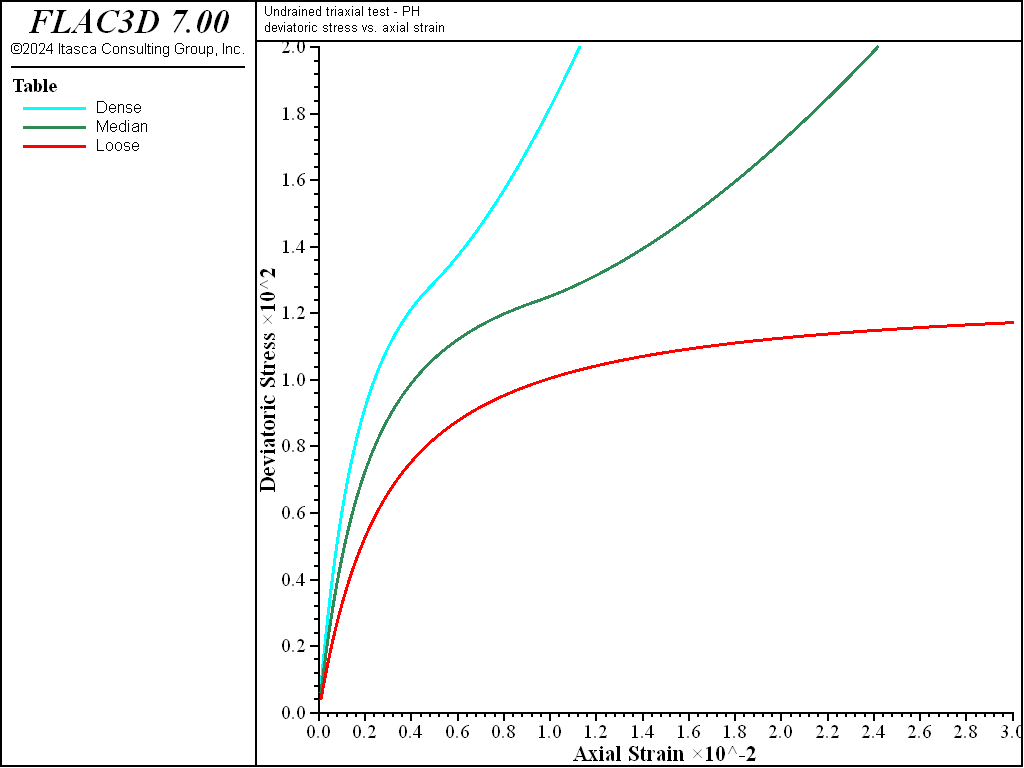FLAC3D Theory and Background • Constitutive Models

# Undrained Triaxial Compression Test with Plastic-Hardening Model

Note

To view this project in FLAC3D, use the menu command Help ► Examples…. Choose “ConstitutiveModels/ UndrainedTriaxialPlasticHardening” and select “UndrainedTriaxialPlasticHardening.prj” to load. The project’s main data file is shown at the end of this example.

Undrained triaxial tests on dense, medium, and loose sand are simulated using the Plastic-Hardening (PH) model are repeated, but this time under undrained conditions. This example does not consider yielding on the cap, therefore the default value $$OCR$$ = 100 is used. The initial stress state is isotropic, with mean pressure equal to 100 kPa. In this example, we realize the undrained condition by setting a zero volumetric strain; therefore, the fluid logic does not need to be configured. Triaxial tests on dense, medium, and loose sand are simulated using the PH model. The model properties are listed in Table 1.

Table 1: Plastic-Hardening Model Properties
Parameter Dense Medium Loose
$$E^{ref}_{50}$$ (kPa) 4.0e4 3.0e4 2.0e4
$$\phi$$ 40 35 30
$$\psi$$ 10 5 0
$$K_{nc}$$ 0.36 0.43 0.50
$$c$$ (kPa)   0
$$p_{ref}$$ (kPa)   100
$$m$$   0.5
$$\sigma^{ini}_{1 \sim 3}$$ (kPa)   -100
$$OCR$$   100
$$e_{max}$$   1.02

The undrained simulation results for deviatoric stress is plotted in Figure 1.Figure 1: $$\mid \sigma_1 - \sigma_3 \mid$$ (in kPa) versus axial strain for dense, medium, and loose sand—undrained triaxial tests.

Data File

UndrainedTriaxialPlasticHardening.dat

; Undrained Triaxial Tests
model large-strain off
model title "Undrained triaxial test - PH"
;
zone create brick size 1 1 1
zone cmodel assign plastic-hardening
;
zone initialize stress xx -100. yy -100. zz -100.
;
zone property density 1000 pressure-ref=100. exponent=0.5 void-maximum 1.02
zone property stiffness-50-ref=[E50ref] stiffness-ur-ref=[3*E50ref] ...
friction=[fric] dilation=[dila] ...
coefficient-normally-consolidation=[knc]
zone property stress-1-effective=-100. stress-2-effective=-100. ...
stress-3-effective=-100.
;
zone gridpoint fix velocity
zone gridpoint fix velocity-z -1e-6 range position-z 1
zone gridpoint fix velocity-x  5e-7 range position-x 1
zone gridpoint fix velocity-y  5e-7 range position-y 1
;
fish define ph_q
return zone.stress.xx(z) - zone.stress.zz(z)
end
;
zone history displacement-z position (1,1,1)
fish history ph_q
history interval 100
;
model step 30000
;
history export '2' vs '1' reverse table 'deviatoricStress'
table 'deviatoricStress' ...
export [string.build('deviatoricStress%1', _IChoice)] truncate